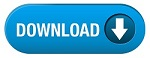Home » SSC » Model Questions for Scientific Assistant (IMD) Exam (Paper-2) PHYSICS – Set 33

# Model Questions for Scientific Assistant (IMD) Exam (Paper-2) PHYSICS – Set 33

## 1. Gauss’s law due to different charge distribution is used to calculate—-

A. electric field
B. electric charge
C. electric intensity
D. electric field lines

A. 1
B. min
C. 0
D. max

A. ohms law
B. newton’s law
C. gauss’s law
D. coulombs law

A. 1
B. 0
C. min
D. max

A. positive
B. negative
C. doubled
D. halved

## 6. If a body is in rest or in uniform velocity, it is said to be in—-

A. rest
B. uniform motion
C. equilibrium
D. constant force

### 7. “Sum of all forces acting on a body is zero”. This condition represents equilibrium’s—-

A. first condition
B. second condition
C. third condition
D. fourth condition

A. positive
B. negative
C. doubled
D. halved

A. AC generator
B. DC generator
C. cell
D. DC motor

A. amperes law
B. Lenz’s law
D. coulombs law

#